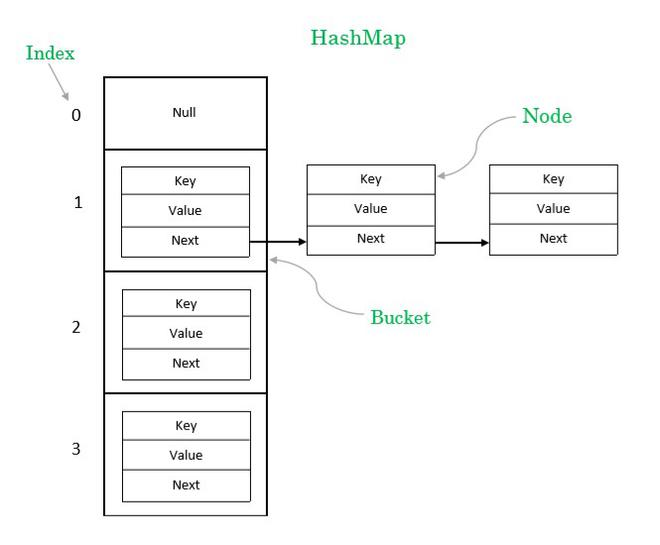# Load Factor in HashMap in Java with Examples

HashMap is a class that implements the Map interface of Java Collections Framework. The most important feature of a HashMap is that it has a constant time performance for retrieval and insertion. The two factors that dictate the performance of a HashMap are:

• Initial Capacity

Before we explain what is the Load Factor of a HashMap, it is essential to understand its structure.

A HashMap has nodes that contain the key value pair much like a node in a Map. HashMap has buckets that contain the nodes, a bucket may contain more than one node. The basic structure of a HashMap is as follows:Schematic of the structure of HashMap

Index: It is the integer value obtained after performing bitwise AND operation on the value of hash of the key and array size minus one.

Index = hashcode(key) & (ArraySize – 1)

where hashcode is a predefined function that returns the integer value of the hash of the key and ArraySize is the number of buckets in the HashMap.

Bucket: It is a LinkedList structure of nodes.

Node: It is the elementary unit of a HashMap. It contains the key-value pair and a link to the next node.

The syntax to declare a HashMap object is as follows:

HashMap objectName = new HashMap(int initialCapacity, float loadFactor)

### Initial Capacity

The Initial Capacity is essentially the number of buckets in the HashMap which by default is 24 = 16. A good HashMap algorithm will distribute an equal number of elements to all the buckets.

Say we have 16 elements then each bucket will have 1 node, the search for any element will be achieved with 1 lookup. If we have 32 elements then each bucket will have 2 nodes, the search for any element will be achieved with 2 lookups. Similarly, if we have 64 elements then each bucket will have 4 nodes, the search for any element will be achieved with 4 lookups and so on.

As you can observe when the number of elements doubles the number of lookup increment by 1, which is good for the performance.

But what happens when the number of elements goes to a very high value, say 10,000, in that case, we will require 625 lookups. Similarly, if the number of elements is 10,00,000 we will require 62,500 lookups. Such a high number of lookups will degrade the performance of the HashMap. This is where the Load Factor comes into play.

The Load Factor is a threshold, if the ratio of the current element by initial capacity crosses this threshold then the capacity increases so that the operational complexity of the HashMap remains O(1). The meaning of operational complexity of O(1) means the retrieval and insertion operations take constant time.
The default load factor of a HashMap is 0.75f.

#### How do we decide when to increase the capacity?

Let us take an example, since the initial capacity by default is 16, consider we have 16 buckets right now.

We insert the first element, the current load factor will be 1/16 = 0.0625. Check is 0.0625 > 0.75 ? The answer is No, therefore we don’t increase the capacity.

Next we insert the second element, the current load factor will be 2/16 = 0.125. Check is 0.125 > 0.75 ? The answer is No, therefore we don’t increase the capacity.

Similarly, for 3rd element, load factor = 3/16 = 0.1875 is not greater than 0.75, No change in the capacity.

4th element, load factor = 4/16 = 0.25 is not greater than 0.75, No change in the capacity.

5th element, load factor = 5/16 = 0.3125 is not greater than 0.75, No change in the capacity.

6th element, load factor = 6/16 = 0.375 is not greater than 0.75, No change in the capacity.

7th element, load factor = 7/16 = 0.4375 is not greater than 0.75, No change in the capacity.

8th element, load factor = 8/16 = 0.5 is not greater than 0.75, No change in the capacity.

9th element, load factor = 9/16 = 0.5625 is not greater than 0.75, No change in the capacity.

10th element, load factor = 10/16 = 0.625 is not greater than 0.75, No change in the capacity.

11th element, load factor = 11/16 = 0.6875 is not greater than 0.75, No change in the capacity.

12th element, load factor = 12/16 = 0.75 is equal to 0.75, still No change in the capacity.

13th element, load factor = 13/16 = 0.8125 is greater than 0.75, at the insertion of the 13th element we double the capacity.

Now the capacity is 32.

In a similar way, every time an insertion crosses the load factor of 0.75 the capacity is doubled for a constant time performance of get() { retrieval } and put() { insertion } operations.

Attention reader! Don’t stop learning now. Get hold of all the important DSA concepts with the DSA Self Paced Course at a student-friendly price and become industry ready.

My Personal Notes arrow_drop_upCheck out this Author's contributed articles.

If you like GeeksforGeeks and would like to contribute, you can also write an article using contribute.geeksforgeeks.org or mail your article to contribute@geeksforgeeks.org. See your article appearing on the GeeksforGeeks main page and help other Geeks.

Please Improve this article if you find anything incorrect by clicking on the "Improve Article" button below.

Article Tags :
Practice Tags :

Be the First to upvote.

Please write to us at contribute@geeksforgeeks.org to report any issue with the above content.